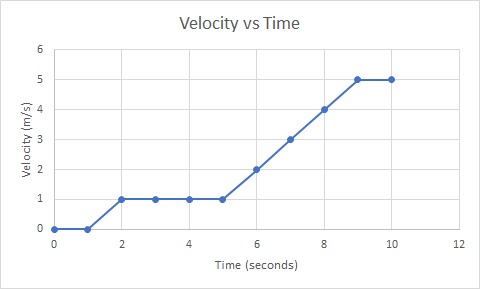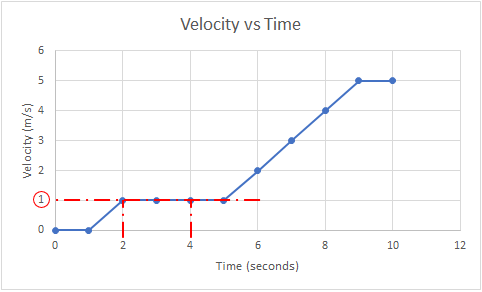## Electron Motion

An electron's motion is tracked and plotted in the shown diagram. What is its acceleration between the 2 and 4 second data points in m/s^2?Hint
Acceleration is defined as:
$$a=\frac{dv}{dt}$$$where $$v$$ is velocity and $$t$$ is time. Hint 2 $$a=\frac{dv}{dt}=\frac{\Delta v}{\Delta t}=\frac{v_{final}-v_{initial}}{t_{final}-t_{initial}}$$$
Acceleration is defined as:
$$a=\frac{dv}{dt}=\frac{\Delta v}{\Delta t}=\frac{v_{final}-v_{initial}}{t_{final}-t_{initial}}$$$where $$v$$ is velocity and $$t$$ is time. The plot’s x-axis is time and y-axis is velocity. Looking at the graph to find the corresponding velocity values associated with 2 and 4 second observation points:Therefore, $$v_{final}=1\:m/s$$ , $$v_{initial}=1\:m/s$$ , $$t_{final}=4\:s$$ , and $$t_{initial}=2\:s$$ . Plugging these values into the acceleration equation: $$a=\frac{1m/s-1m/s}{4s-2s}=\frac{0m/s}{2s}=0\:m/s^2$$$
$$0\:m/s^2$$\$